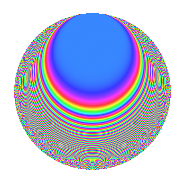# Properties

 Label 1144.2.aLevel 1144 Weight 2 Character orbit a Rep. character $$\chi_{1144}(1,\cdot)$$ Character field $$\Q$$ Dimension 30 Newforms 12 Sturm bound 336 Trace bound 13

# Related objects

## Defining parameters

 Level: $$N$$ = $$1144 = 2^{3} \cdot 11 \cdot 13$$ Weight: $$k$$ = $$2$$ Character orbit: $$[\chi]$$ = 1144.a (trivial) Character field: $$\Q$$ Newforms: $$12$$ Sturm bound: $$336$$ Trace bound: $$13$$ Distinguishing $$T_p$$: $$3$$, $$5$$, $$7$$, $$17$$

## Dimensions

The following table gives the dimensions of various subspaces of $$M_{2}(\Gamma_0(1144))$$.

Total New Old
Modular forms 176 30 146
Cusp forms 161 30 131
Eisenstein series 15 0 15

The following table gives the dimensions of the cuspidal new subspaces with specified eigenvalues for the Atkin-Lehner operators and the Fricke involution.

$$2$$$$11$$$$13$$FrickeDim.
$$+$$$$+$$$$+$$$$+$$$$3$$
$$+$$$$+$$$$-$$$$-$$$$3$$
$$+$$$$-$$$$+$$$$-$$$$6$$
$$+$$$$-$$$$-$$$$+$$$$3$$
$$-$$$$+$$$$+$$$$-$$$$3$$
$$-$$$$+$$$$-$$$$+$$$$3$$
$$-$$$$-$$$$+$$$$+$$$$3$$
$$-$$$$-$$$$-$$$$-$$$$6$$
Plus space$$+$$$$12$$
Minus space$$-$$$$18$$

## Trace form

 $$30q$$ $$\mathstrut -\mathstrut 4q^{5}$$ $$\mathstrut +\mathstrut 22q^{9}$$ $$\mathstrut +\mathstrut O(q^{10})$$ $$30q$$ $$\mathstrut -\mathstrut 4q^{5}$$ $$\mathstrut +\mathstrut 22q^{9}$$ $$\mathstrut +\mathstrut 6q^{11}$$ $$\mathstrut +\mathstrut 12q^{15}$$ $$\mathstrut +\mathstrut 16q^{21}$$ $$\mathstrut +\mathstrut 30q^{25}$$ $$\mathstrut +\mathstrut 24q^{29}$$ $$\mathstrut -\mathstrut 4q^{33}$$ $$\mathstrut -\mathstrut 28q^{35}$$ $$\mathstrut -\mathstrut 12q^{37}$$ $$\mathstrut -\mathstrut 16q^{41}$$ $$\mathstrut -\mathstrut 16q^{43}$$ $$\mathstrut +\mathstrut 24q^{45}$$ $$\mathstrut +\mathstrut 12q^{47}$$ $$\mathstrut -\mathstrut 2q^{49}$$ $$\mathstrut -\mathstrut 12q^{51}$$ $$\mathstrut +\mathstrut 4q^{53}$$ $$\mathstrut +\mathstrut 12q^{59}$$ $$\mathstrut -\mathstrut 24q^{61}$$ $$\mathstrut +\mathstrut 72q^{63}$$ $$\mathstrut +\mathstrut 4q^{67}$$ $$\mathstrut +\mathstrut 20q^{69}$$ $$\mathstrut +\mathstrut 8q^{71}$$ $$\mathstrut -\mathstrut 8q^{73}$$ $$\mathstrut +\mathstrut 72q^{75}$$ $$\mathstrut -\mathstrut 24q^{79}$$ $$\mathstrut +\mathstrut 30q^{81}$$ $$\mathstrut -\mathstrut 48q^{87}$$ $$\mathstrut +\mathstrut 12q^{91}$$ $$\mathstrut -\mathstrut 44q^{93}$$ $$\mathstrut +\mathstrut 40q^{95}$$ $$\mathstrut -\mathstrut 32q^{97}$$ $$\mathstrut +\mathstrut 18q^{99}$$ $$\mathstrut +\mathstrut O(q^{100})$$

## Decomposition of $$S_{2}^{\mathrm{new}}(\Gamma_0(1144))$$ into irreducible Hecke orbits

Label Dim. $$A$$ Field CM Traces A-L signs $q$-expansion
$$a_2$$ $$a_3$$ $$a_5$$ $$a_7$$ 2 11 13
1144.2.a.a $$1$$ $$9.135$$ $$\Q$$ None $$0$$ $$1$$ $$-1$$ $$-2$$ $$-$$ $$-$$ $$+$$ $$q+q^{3}-q^{5}-2q^{7}-2q^{9}+q^{11}-q^{13}+\cdots$$
1144.2.a.b $$1$$ $$9.135$$ $$\Q$$ None $$0$$ $$1$$ $$-1$$ $$-2$$ $$+$$ $$-$$ $$-$$ $$q+q^{3}-q^{5}-2q^{7}-2q^{9}+q^{11}+q^{13}+\cdots$$
1144.2.a.c $$1$$ $$9.135$$ $$\Q$$ None $$0$$ $$2$$ $$3$$ $$1$$ $$-$$ $$+$$ $$+$$ $$q+2q^{3}+3q^{5}+q^{7}+q^{9}-q^{11}-q^{13}+\cdots$$
1144.2.a.d $$1$$ $$9.135$$ $$\Q$$ None $$0$$ $$3$$ $$3$$ $$-3$$ $$+$$ $$-$$ $$+$$ $$q+3q^{3}+3q^{5}-3q^{7}+6q^{9}+q^{11}+\cdots$$
1144.2.a.e $$2$$ $$9.135$$ $$\Q(\sqrt{5})$$ None $$0$$ $$-3$$ $$-2$$ $$-5$$ $$-$$ $$+$$ $$+$$ $$q+(-1-\beta )q^{3}+(-2+2\beta )q^{5}+(-2+\cdots)q^{7}+\cdots$$
1144.2.a.f $$2$$ $$9.135$$ $$\Q(\sqrt{5})$$ None $$0$$ $$-3$$ $$-2$$ $$1$$ $$-$$ $$-$$ $$+$$ $$q+(-1-\beta )q^{3}-2\beta q^{5}+\beta q^{7}+(-1+\cdots)q^{9}+\cdots$$
1144.2.a.g $$2$$ $$9.135$$ $$\Q(\sqrt{5})$$ None $$0$$ $$-3$$ $$-2$$ $$1$$ $$+$$ $$-$$ $$-$$ $$q+(-1-\beta )q^{3}+(-2+2\beta )q^{5}+(2-3\beta )q^{7}+\cdots$$
1144.2.a.h $$3$$ $$9.135$$ 3.3.229.1 None $$0$$ $$0$$ $$-5$$ $$2$$ $$-$$ $$+$$ $$-$$ $$q-\beta _{1}q^{3}+(-2+\beta _{1}-\beta _{2})q^{5}+(1+\beta _{2})q^{7}+\cdots$$
1144.2.a.i $$3$$ $$9.135$$ 3.3.229.1 None $$0$$ $$0$$ $$1$$ $$-4$$ $$+$$ $$+$$ $$+$$ $$q-\beta _{1}q^{3}+(\beta _{1}-\beta _{2})q^{5}+(-1+\beta _{2})q^{7}+\cdots$$
1144.2.a.j $$3$$ $$9.135$$ 3.3.229.1 None $$0$$ $$3$$ $$1$$ $$6$$ $$+$$ $$+$$ $$-$$ $$q+(1+\beta _{1})q^{3}+(\beta _{1}-\beta _{2})q^{5}+(2+\beta _{1}+\cdots)q^{7}+\cdots$$
1144.2.a.k $$5$$ $$9.135$$ 5.5.7698829.1 None $$0$$ $$0$$ $$-4$$ $$6$$ $$+$$ $$-$$ $$+$$ $$q+\beta _{1}q^{3}+(-1-\beta _{3})q^{5}+(1+\beta _{2}-\beta _{3}+\cdots)q^{7}+\cdots$$
1144.2.a.l $$6$$ $$9.135$$ $$\mathbb{Q}[x]/(x^{6} - \cdots)$$ None $$0$$ $$-1$$ $$5$$ $$-1$$ $$-$$ $$-$$ $$-$$ $$q-\beta _{1}q^{3}+(1-\beta _{2})q^{5}+\beta _{5}q^{7}+(2+\beta _{4}+\cdots)q^{9}+\cdots$$

## Decomposition of $$S_{2}^{\mathrm{old}}(\Gamma_0(1144))$$ into lower level spaces

$$S_{2}^{\mathrm{old}}(\Gamma_0(1144)) \cong$$ $$S_{2}^{\mathrm{new}}(\Gamma_0(11))$$$$^{\oplus 8}$$$$\oplus$$$$S_{2}^{\mathrm{new}}(\Gamma_0(26))$$$$^{\oplus 6}$$$$\oplus$$$$S_{2}^{\mathrm{new}}(\Gamma_0(44))$$$$^{\oplus 4}$$$$\oplus$$$$S_{2}^{\mathrm{new}}(\Gamma_0(52))$$$$^{\oplus 4}$$$$\oplus$$$$S_{2}^{\mathrm{new}}(\Gamma_0(88))$$$$^{\oplus 2}$$$$\oplus$$$$S_{2}^{\mathrm{new}}(\Gamma_0(104))$$$$^{\oplus 2}$$$$\oplus$$$$S_{2}^{\mathrm{new}}(\Gamma_0(143))$$$$^{\oplus 4}$$$$\oplus$$$$S_{2}^{\mathrm{new}}(\Gamma_0(286))$$$$^{\oplus 3}$$$$\oplus$$$$S_{2}^{\mathrm{new}}(\Gamma_0(572))$$$$^{\oplus 2}$$